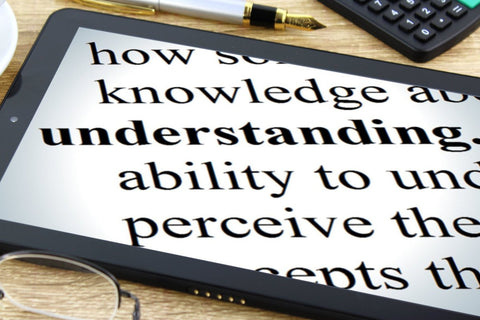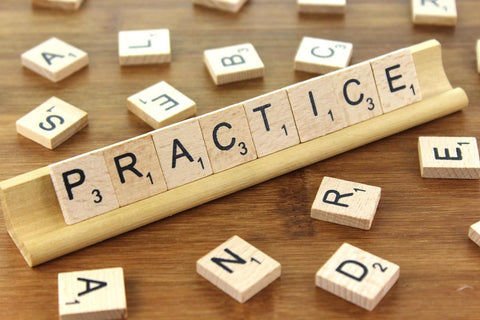# Learning Mathematics is EASY## Learning Mathematics is EASY as 1 2 3

Authored By Carmen Popescu-Rose 1 Comment(s)

Yes ... you read it correctly: Learning Mathematics is EASY as 1 2 3.

STEP 1 Understand the topic.Many students think that if they have trouble understanding a mathematical topic or skill, this means that they cannot do maths. This cannot be further from the truth. There are topics that are hard to get your head around and there are topics that are easy to understand straight away. This means that you need more explanations or you might need someone to explain that topic to you in a different way.

What to do then ...?

• ask a friend to explain it to you;
• ask a teacher to explain it to you in a different way;
• watch a few videos (so many available out there) that explain it in different ways;
• try to explain what you understand to a friend;
• take a break to clear your mind and try again later.

STEP 2 Work out some examples.Examples are a great way to help you understand a topic and practise a mathematical skill you are learning. Many students just look at examples or read examples and have trouble understanding the steps required to complete them.

What to do then ...?

• grab a pen and paper and actually follow the steps in the example by writing them down;
• try to understand each step;
• ask yourself: why do I have to do this step?;
• discuss the example with a friend;
• do more than one example.

STEP 3 Practice makes perfect.Many students think that, if they understand what they are doing and can do a couple of examples, then they do not have to practise anymore. Well ... it doesn't always work like this. Quite often, a concept or a skill that is not practised enough gets lost. Practice is needed to move what you have learnt from the short term memory into the long term memory.

What to do then ...?

• do as many questions as needed to change you from a novice to a master of the topic or skill you are learning.
This is all it takes to learn Mathematics. Isn't this easy!?